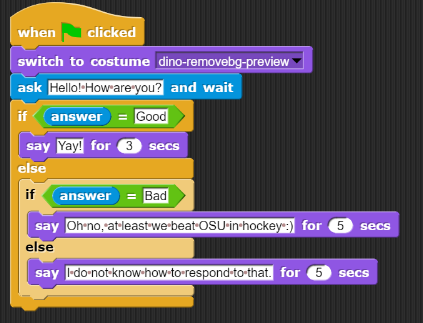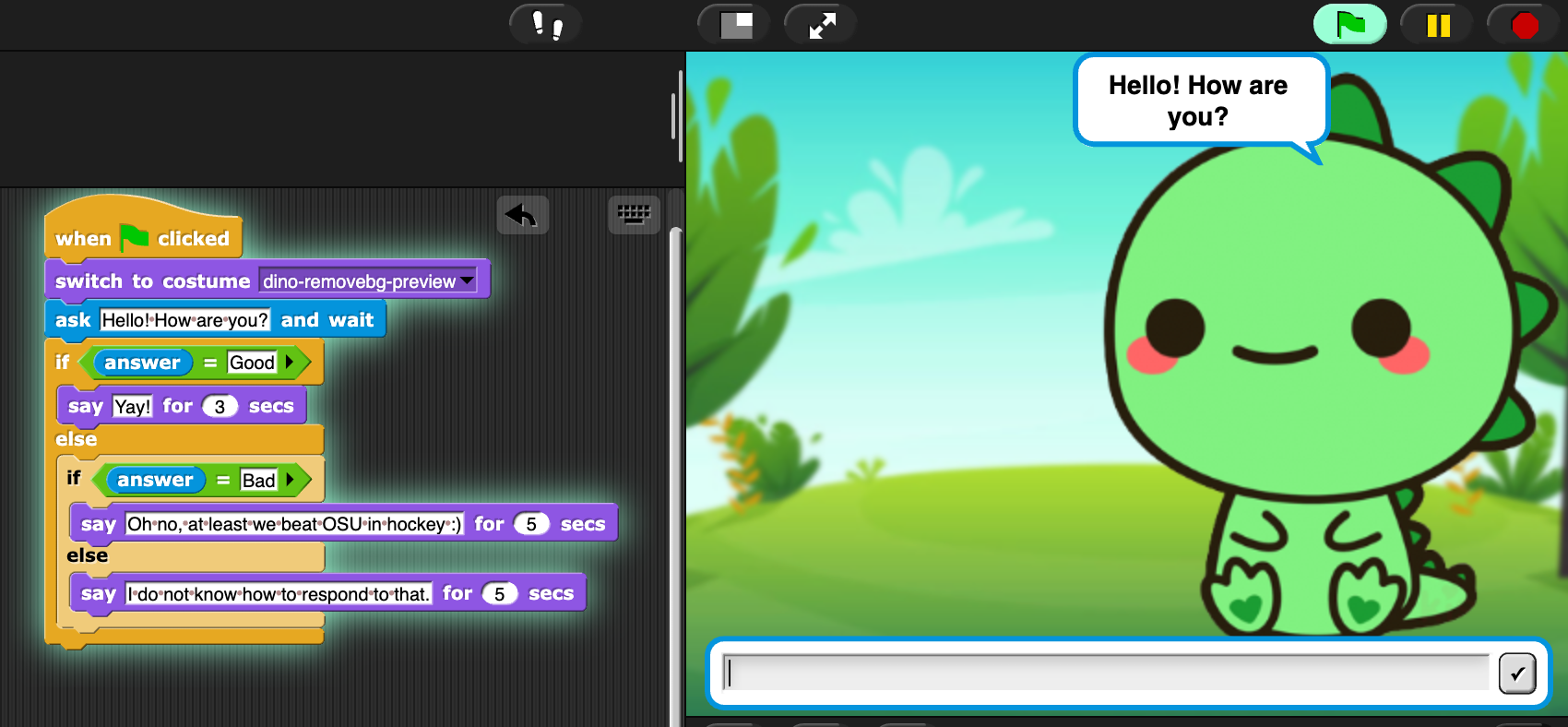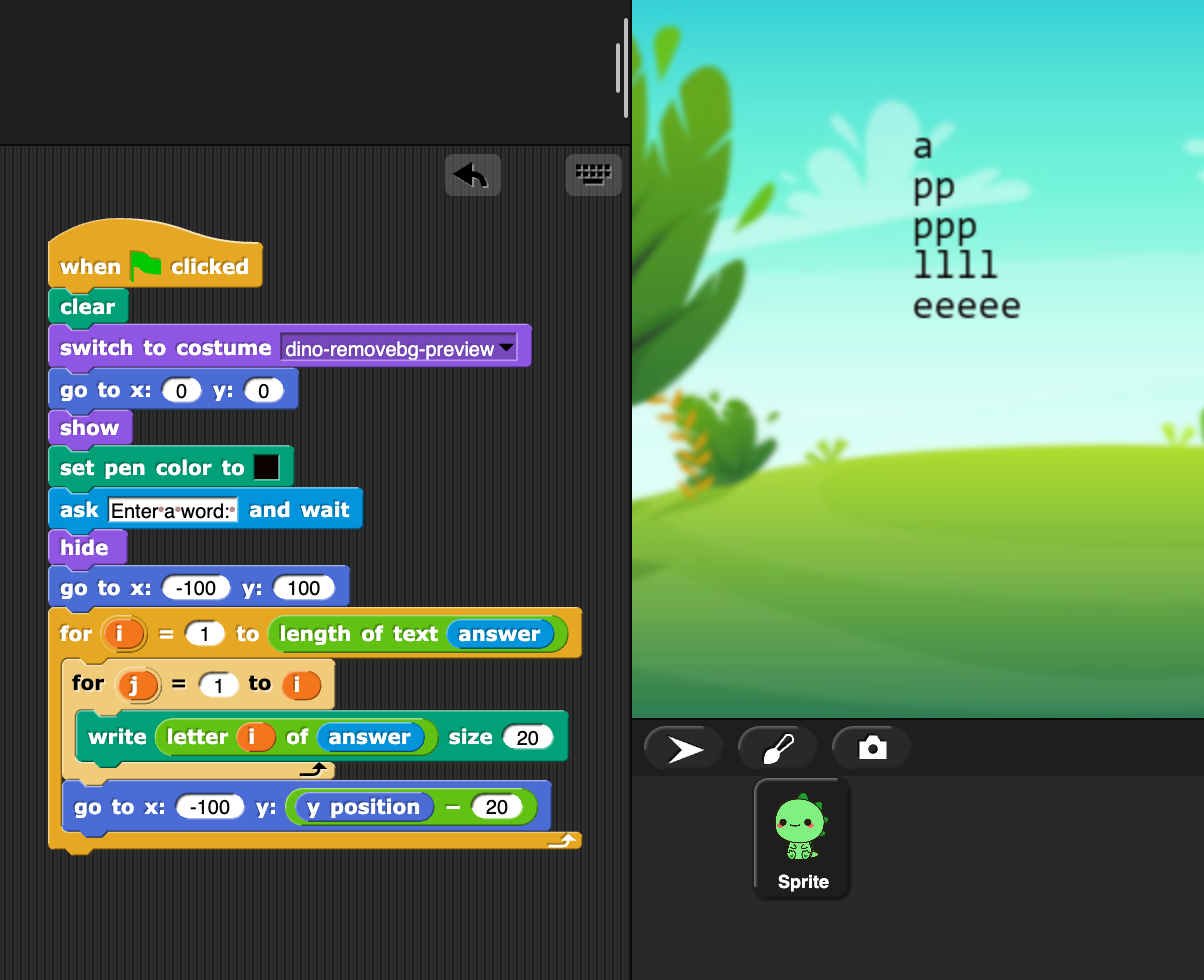# Chapter 4: Conditionals and Loops¶

Chapter written by Chloe Nguyen

In the previous chapters, you have explored how to map from Snap code into Python, SQL, and HTML code. The idea is to make sense of textual programming language based on what you know from Snap.

In this chapter, we reverse the process. We are going to start with two key ideas in programming languages (textual or block-based): Conditionals and loops. We use both Snap and Python to provide examples.

## Section 1: Conditionals¶

Conditionals are also known as if clauses and we use them all the time to express that one thing depends on something else. This idea transfers to programming.

Let’s start with the “if” statement. If you ask someone how their day is, your answer depends on their response. You wouldn’t say “Yay!” if someone told you that they had a bad day (hopefully). You would only say “Yay!” if someone says they are “Good.”

Then there is the “else if” statement. Let’s say you checked for “Good,” but did not find it. Give yourself another option! Check for “Bad,” which you could answer with something positive (e.g. “Oh no, at least we beat OSU in hockey”).

Finally, there is the “else” clause. You check and the answer is not “Good” or “Bad,” so you make a “catch-all” answer for any other response.

### The Snap Version¶Here is a simple dialog project that shows the logic from above. Here’s what it looks when running.### The Python Version¶

The below program does the same thing in Python. Click ‘Run’ to have a conversation.

Let’s look at this syntax. The first if statment is checking to see if the word “Good’ is in the answer, which was input from the user. “Elif” stands for else if and checks for “Bad” in the answer as long as “Good” was not found. Finally, “else” tells the user that the computer has no idea how to respond to what the user inputted.

### Another Example¶

Do you know when you sign up for a new account and have to enter your birthday? Some websites have you put a number for your month, others give you a drop down menu, and still others show a calender. Here is a simple Snap project for converting months that takes in a number and gives your birth month. Note that instead of an “else if” statement, Snap just has you put an if statement inside of another one. It works the same way! The first if statement is checked before the second one, the second before the third, and so on.

The below program does the same thing in Python. Click ‘Run’ to try it out.

## Section 2: Loops¶

Loops are exactly what they sound like, doing the same thing again and again. There are several different types of loops, but we are going to focus on the for loop. A for loop is used when you know how many times you have to do something.

For example, imagine making a circle in Snap. You would move a few steps forward and then turn. And then repeat that a bunch of times. Let’s say you turned 1 degree every time, you would repeat the process 360 times to get a circle!

### The Snap Version¶Here is a project that takes in a word from the user and makes a right triangle out of the letters! Try “apple” as in this example, or another word like “dinosaur.”

This program has two loops. The one counts i from 1 to the length (in characters) of the input answer. The interior loop counts j from 1 to the value of i. Inside that, we write the letters of the answer. So the first time through the loop, i is 1 and j is 1, so we print just one letter. In the second time, i is 2 and j is 1 and then 2. Third time, i is 3 and j is 1, then 2, then 3. We use j to reference the character that gets written on the screen.

### The Python Version¶

Now this code does not do the exact same thing yet. Let’s walk through it
• takes in a word from the user, e.g. apple

• finds the length of the word, e.g. 5

• makes a for loop that runs 5 times

• outputs word[i]

How do we know that we loop around 5 times? The line “for i in range(length):” creates a for loop that goes around “length” number of times doing whatever is after the colon. We start with that variable i equal to 0 and print something out. The next time around, i = 1 and something is printed out (and so on).

If the word is apple, length is equal to 5. The for loop goes until the value of i is equal to or greater to whatever number is inside range(). With that logic, the loop STOPS when i goes to 5. That means it outputs something for i = 0, 1, 2, 3, 4. Or, in other words, goes around 5 times.

What are we printing? The variable “word” is the word that the user gave us. The phrase “word[i]” gives us a letter from the word. If i = 0, it would be ‘a.’ If i = 1, it would be ‘p.’ If i = 2, it would be ‘p.’ If i = 3, it would be ‘l.’ If i = 4, it would be ‘e.’

Here is the Python code that matches the Snap project above.

Now there are two for loops and we have our right triangle. Here a few hints about how this works:
• once the first for loop is entered, the second for loop is entered and runs COMPLETELY (j = 1, j = 2, j = 3,…) before the first for loop “loops”

• i changes every time! that means the number of times the second for loop “loops” also changes every time

• when i = 0, j runs once

• when i = 1, j runs twice